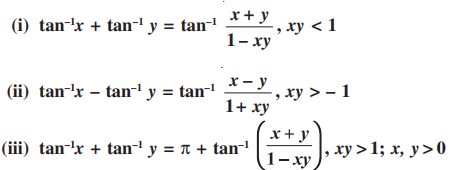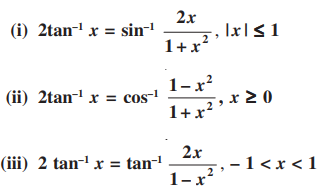# Inverse Trigonometric Functions For Class 12

Inverse Trigonometric Functions for Class 12 includes the major concepts related to the inverse of trigonometric functions, which will help the students score good marks in their examinations. The inverse trigonometric functions play an essential role in calculus, for they serve to define many integrals. The concepts of inverse trigonometric functions are also used in science and engineering.

In this chapter, you will learn about the restrictions on domains and ranges of trigonometric functions, which assure the existence of their inverses and observe their behaviour through graphical representations. Besides, students can also learn some elementary properties of inverse trigonometric functions.

Click here to get the Notes for all chapters of Class 12 Maths.

## Inverse Trigonometric Functions Class 12 Concepts

The concepts covered in this Class 12 Chapter 2 Inverse Trigonometric Functions are:

• Introduction
• Basic Concepts
• Properties of Inverse Trigonometric Functions

## Inverse Trigonometric Functions Class 12 Notes

Basics Concepts

Let’s recall the domain and range of trigonometric functions.

sine function, i.e., sine : R → [– 1, 1]

cosine function, i.e., cos : R → [– 1, 1]

tangent function, i.e., tan : R – { x : x = (2n + 1)π/2, n ∈ Z} →R

cotangent function, i.e., cot : R – { x : x = nπ, n ∈ Z} →R

secant function, i.e., sec : R – { x : x = (2n + 1)π/2, n ∈ Z} →R – (– 1, 1)

cosecant function, i.e., cosec : R – { x : x = nπ, n ∈ Z} →R – (– 1, 1)

The below table gives the inverse trigonometric function (principal value branches) along with their domains and ranges.

 Function Name Notation Definition Domain of x Range Arcsine or inverse sine y = sin-1(x) x = sin y −1 ≤ x ≤ 1 i.e. [-1, 1] − π/2 ≤ y ≤ π/2 -90°≤ y ≤ 90° Arccosine or inverse cosine y = cos-1(x) x = cos y −1 ≤ x ≤ 1 i.e. [-1, 1] 0 ≤ y ≤ π 0° ≤ y ≤ 180° Arctangent or Inverse tangent y = tan-1(x) x = tan y For all real numbers − π/2 < y < π/2 -90°< y < 90° Arccotangent or Inverse Cot y = cot-1(x) x = cot y For all real numbers 0 < y < π 0° < y < 180° Arcsecant or Inverse Secant y = sec-1(x) x = sec y x ≤ −1 or 1 ≤ x R – (-1, 1) 0 ≤ y < π/2 or π/2 < y ≤ π 0° ≤ y < 90° or 90° < y ≤ 180° Arccosecant y = csc-1(x) x = csc y x ≤ −1 or 1 ≤ x R – (-1, 1) −π/2 ≤ y < 0 or 0 < y ≤ π/2 −90° ≤ y < 0° or 0° < y ≤ 90°

### Properties of Inverse Trigonometric Functions

Some properties of inverse trigonometric functions are listed below:

Set 1:

sin−1(1/x) = cosec−1x, x ≥ 1 or x ≤ −1

cos−1(1/x) = sec−1x, x ≥ 1 or x ≤ −1

tan−1(1/x) = cot–1x, x > 0

Set 2:

(i) sin–1(–x) = – sin–1 x, x ∈ [– 1, 1]

(ii) tan–1(–x) = – tan–1 x, x ∈ R

(iii) cosec–1(–x) = – cosec–1 x, | x | ≥ 1

Set 3:

(i) cos–1(–x) = π – cos–1 x, x ∈ [– 1, 1]

(ii) sec–1(–x) = π – sec–1 x, | x | ≥ 1

(iii) cot–1(–x) = π – cot–1 x, x ∈ R

Set 4:

(i) sin–1 x + cos–1 x = π/2, x ∈ [– 1, 1]

(ii) tan–1 x + cot–1 x = π/2, x ∈ R

(iii) cosec–1 x + sec–1 x = π/2, |x| ≥ 1

Set 5:Set 6: